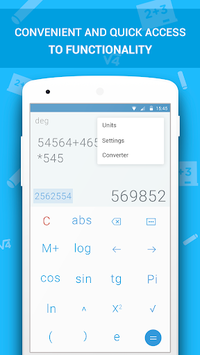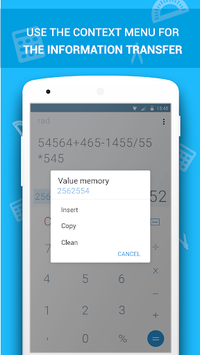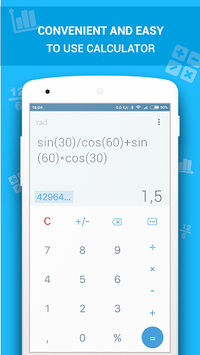# Math Calculator APK

This advanced calculator app quickly provides you with all math computation!

### APK Details

 Category Education Last Updated 2018-08-10 Latest Version 1.5 Size 7.97 MB Installations 100000+ Android version 4.1 and up Content Rating Everyone

### Screenshots## Math Calculator APK Description

Didn't you know that most of your math problems could be solved with one of Android apps free? This highly useful and efficient calculator app will become your best friend at work, in everyday life, at school, college or wherever you study.

Do you love calculators which can help you solve really complex problems? Do you want to get results quickly? Install Math Calculator for free and you'll get a real math problem solver, conversion calculators option as well as many other bonuses.

What do you want to have in case in you don't have math skills at all, but have to solve a really hard problem immediately? Of course, you'd like to have a math calculator that shows work and do it perfectly!

See below the advantages of one of the most exceptional calculators free:

- wide field of application to calculate completely different values and solve various difficult problems
- suitable for all ages and math proficiency
- simple and intuitive design
- haptic feedback (on/off)
- unit converter (distance, weight, speed, time)
- work with different numeral systems
- work with memory register of the calculator (m+, m-, mr, mc)

This calculator covers the following areas of mathematics:

- simple arithmetic (addition, subtraction, multiplication, division, percent)
- elementary algebra (exponentiation, root extraction, decimal and natural logarithms, sin, cos, tan etc)
- elementary functions (logarithmic, trigonometric, power functions)
- derivatives
- integral calculus
- factorial estimation
- equations (linear, quadratic, system of linear equations)
- matrices (conjugation of matrices, determinant of matrix, multiplication of matrices/ matrices with numbers, matrix rank estimation, inverse matrix definition)

As you see, this app is not only a math calculator for algebra problems or basic computation, it is appropriate for everybody: from a kid to an engineer! Don't leave your problems unsolved, install Math Calculator right now!